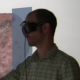# Small Correction to Rift’s Projection Matrix

In a previous post, I looked at the Oculus Rift’s internal projection in detail, and did some analysis of how stereo rendering setup is explained in the Rift SDK’s documentation. Looking at that again, I noticed something strange.

In the other post, I simplified the Rift’s projection matrix as presented in the SDK documentation to$P = \begin{pmatrix} \frac{2 \cdot \mathrm{EyeToScreenDistance}}{\mathrm{HScreenSize} / 2} & 0 & 0 & 0 \\ 0 & \frac{2 \cdot \mathrm{EyeToScreenDistance}}{\mathrm{VScreenSize}} & 0 & 0 \\ 0 & 0 & \frac{z_\mathrm{far}}{z_\mathrm{near} - z_\mathrm{far}} & \frac{z_\mathrm{far} \cdot z_\mathrm{near}}{z_\mathrm{near} - z_\mathrm{far}} \\ 0 & 0 & -1 & 0 \end{pmatrix}$

which, to those in the know, doesn’t look like a regular OpenGL projection matrix, such as created by glFrustum(…). More precisely, the third row of P is off. The third-column entry should be$\frac{z_\mathrm{near} + z_\mathrm{far}}{z_\mathrm{near} - z_\mathrm{far}}$ instead of$\frac{z_\mathrm{far}}{z_\mathrm{near} - z_\mathrm{far}}$, and the fourth-column entry should be$2 \cdot \frac{z_\mathrm{far} \cdot z_\mathrm{near}}{z_\mathrm{near} - z_\mathrm{far}}$ instead of$\frac{z_\mathrm{far} \cdot z_\mathrm{near}}{z_\mathrm{near} - z_\mathrm{far}}$. To clarify, I didn’t make a mistake in the derivation; the matrix’s third row is the same in the SDK documentation.

What’s the difference? It’s subtle. Changing the third row of the projection matrix doesn’t change where pixels end up on the screen (that’s the good news). It only changes the z, or depth, value assigned to those pixels. In a standard OpenGL frustum matrix, 3D points on the near plane get a depth value of 1.0, and those on the far plane get a depth value of -1.0. The 3D clipping operation that’s applied to any triangle after projection uses those depth values to cut off geometry outside the view frustum, and the viewport projection after that will map the [-1.0, 1.0] depth range to [0, 1] for z-buffer hidden surface removal.

Using a projection matrix as presented in the previous post, or in the SDK documentation, will still assign a depth value of -1.0 to points on the far plane, but a depth value of 0.0 to points on the (nominal) near plane. Meaning that the near plane distance given as parameter to the matrix is not the actual near plane distance used by clipping and z buffering, which might lead to some geometry appearing in the view that shouldn’t, and a loss of resolution in the z buffer because only half the value range is used.

I’m assuming that this is just a typo in the Oculus SDK documentation, and that the library code does the right thing (I haven’t looked).

Oh, right, so the fixed projection matrix, for those working along, is$P = \begin{pmatrix} \frac{2 \cdot \mathrm{EyeToScreenDistance}}{\mathrm{HScreenSize} / 2} & 0 & 0 & 0 \\ 0 & \frac{2 \cdot \mathrm{EyeToScreenDistance}}{\mathrm{VScreenSize}} & 0 & 0 \\ 0 & 0 & \frac{z_\mathrm{near} + z_\mathrm{far}}{z_\mathrm{near} - z_\mathrm{far}} & 2 \cdot \frac{z_\mathrm{far} \cdot z_\mathrm{near}}{z_\mathrm{near} - z_\mathrm{far}} \\ 0 & 0 & -1 & 0 \end{pmatrix}$

## 3 thoughts on “Small Correction to Rift’s Projection Matrix”

1.yuriks on said:

Direct3D uses the convention that Z is in [0,1], without the extra rescaling step. This allows for better use of depth buffer precision, and the same can be done in OpenGL using that projection matrix and a vendor extension. You can read about it here: http://outerra.blogspot.com.br/2012/11/maximizing-depth-buffer-range-and.html

•okreylos on said:

Well, shows you I haven’t really thought about z buffer precision in much detail. Thanks for pointing that out, and thanks for the link. Good reading!